# Rust Stable 1.45 中的 神奇 Bug 解读

2020/07/26 17:43Bug 描述

#74739
https://github.com/rust-lang/rust/issues/74739

struct Foo {    x: i32,}fn main() {    let mut foo = Foo { x: 42 };    let x = &mut foo.x;    *x = 13;    let y = foo;    println!("{}", y.x); // -> 42; expected result: 13}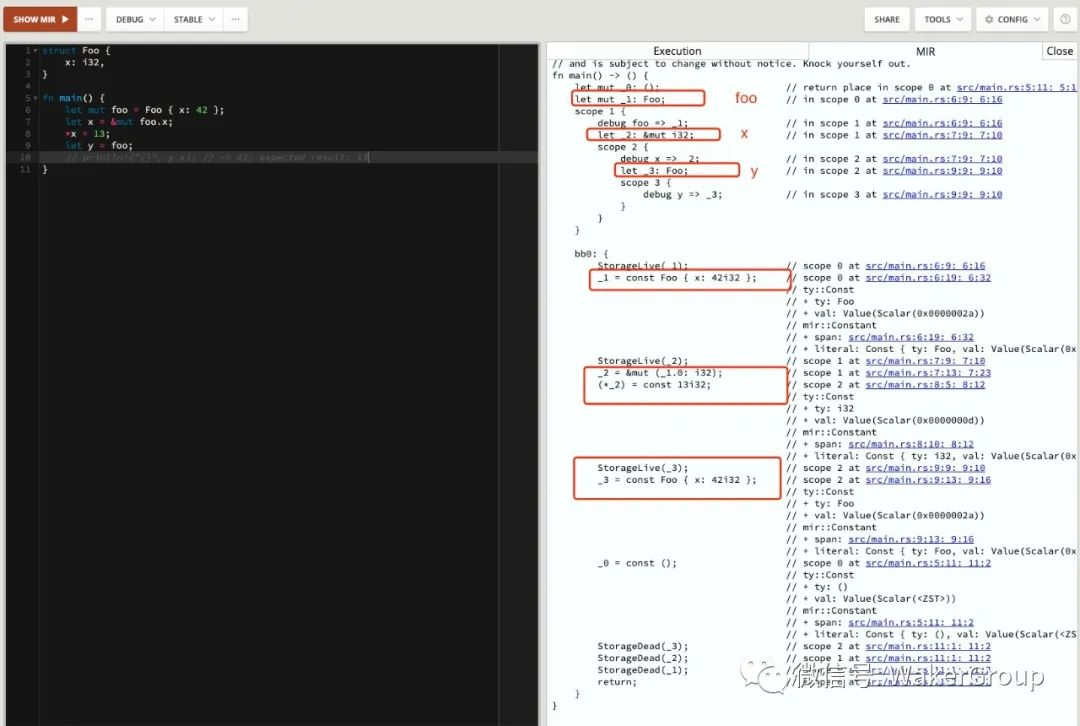_1 = const Foo { x: 42i32 };...(*_2) = const 13i32;  ..._3 = const Foo { x: 42i32 };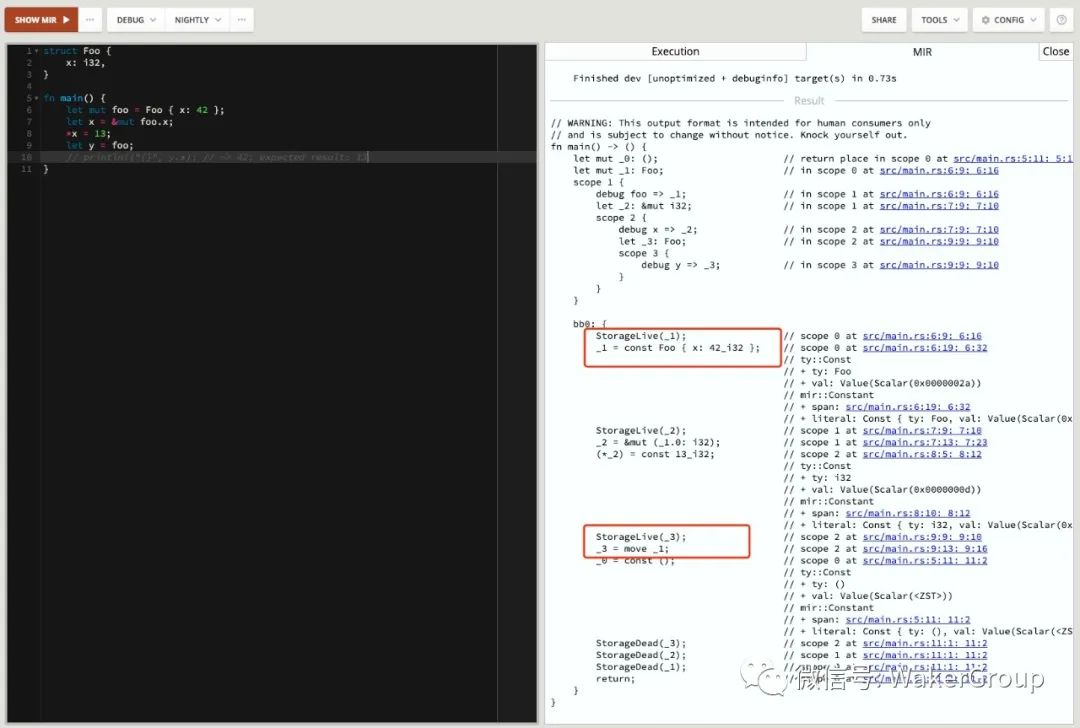_1 = const Foo { x: 42i32 };...(*_2) = const 13i32;  ..._3 = move _1;

#[derive(Copy, Clone)]struct Foo {    x: i32,}fn main() {    let mut foo = Foo { x: 42 };    let x = &mut foo.x;    *x = 13;    let y = foo;    println!("{}", y.x); // -> 42; expected result: 13}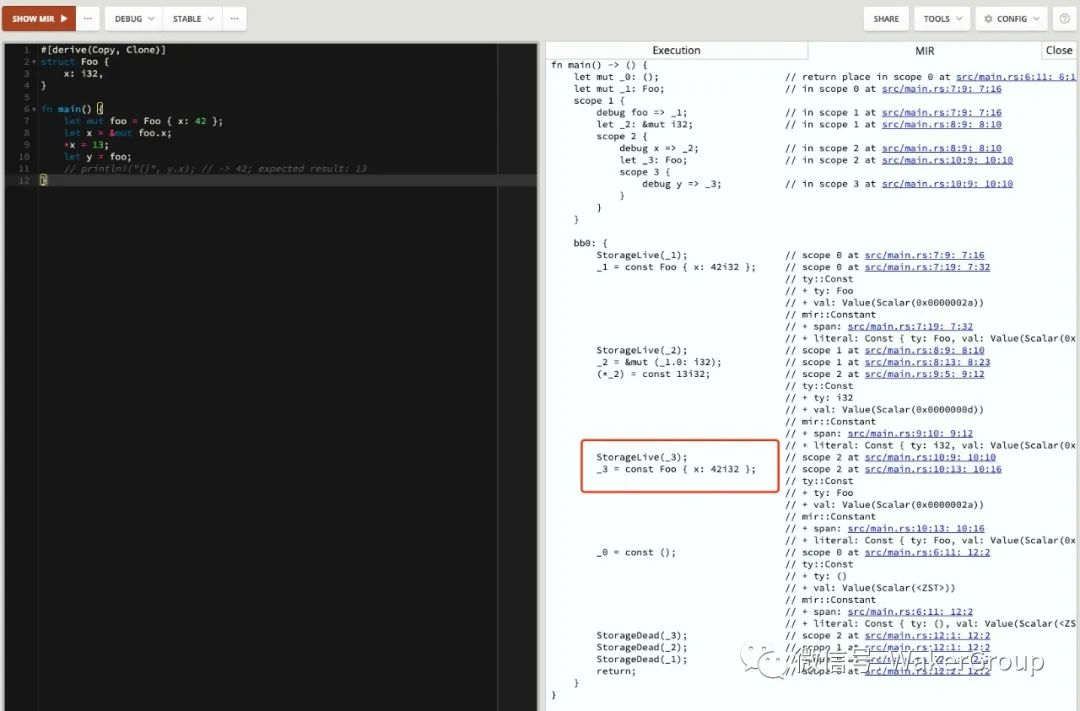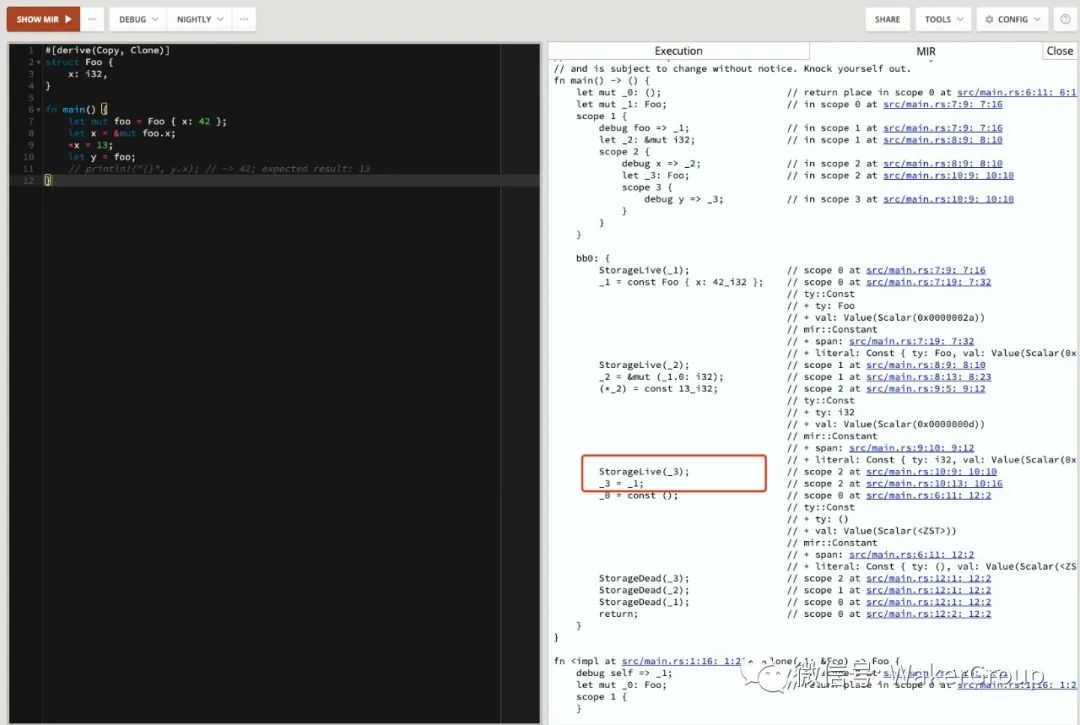Bug 产生根源

_1 = const Foo { x: 42i32 };...(*_2) = const 13i32;  ..._3 = move _1;// 或者_3 = _1;

_1 = const Foo { x: 42i32 };...(*_2) = const 13i32;  ..._3 = const Foo { x: 42i32 };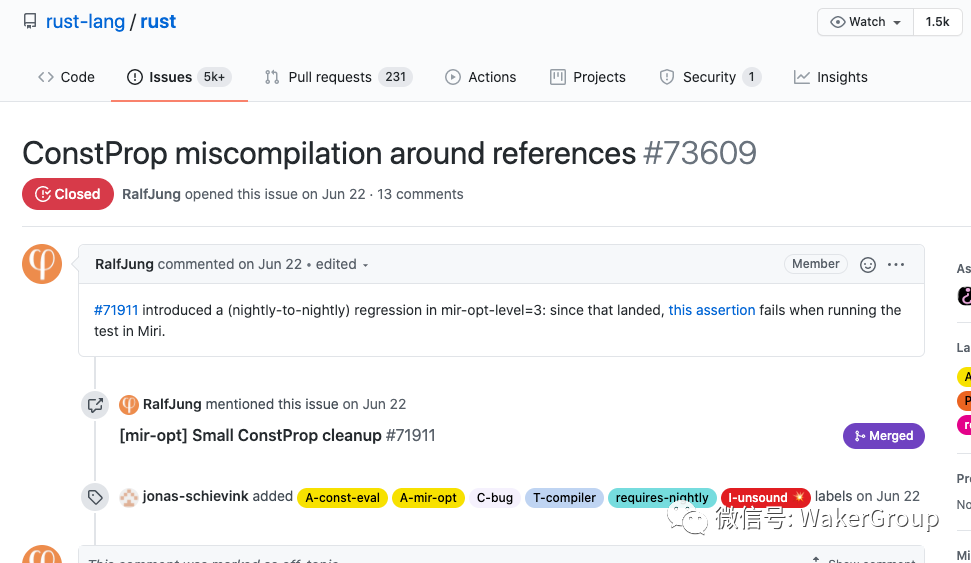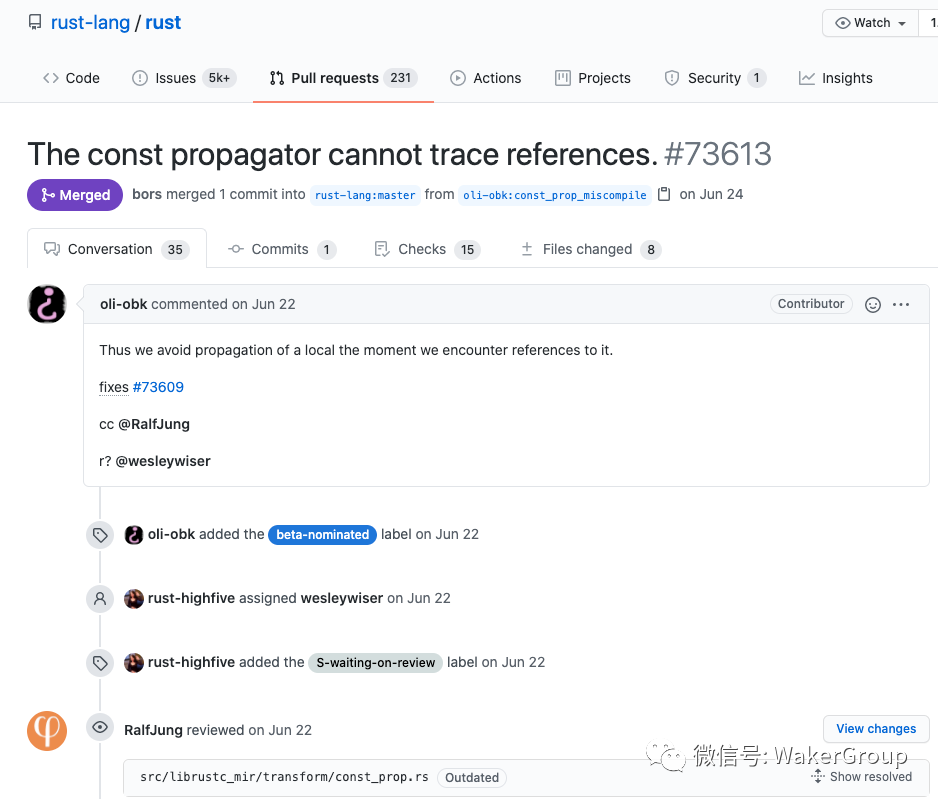_1 = const Foo { x: 42i32 };...(*_2) = const 13i32;  ..._3 = const Foo { x: 42i32 };

Rust 官方在 2019年12月初公布了编译器引入了常量传播技术，用来优化 MIR 。相关链接：https://blog.rust-lang.org/inside-rust/2019/12/02/const-prop-on-by-default.html

1. 编译器可以自动识别代码中的常量，即便你没有显式声明。比如下面代码所示：

struct Point {  x: u32,  y: u32,}let a = 2 + 2; // optimizes to 4let b = [0, 1, 2, 3, 4, 5]; // optimizes to 3let c = (Point { x: 21, y: 42 }).y; // optimizes to 42

2. 消除控制流。比如下面代码所示：

const Foo: Option<u8> = Some(12);let x = match Foo {   None => panic!("no value"),   Some(v) => v,};// 优化为const Foo: Option<u8> = Some(12);let x = 12;

let x = 2 + 4 * 6;

let (_tmp0, overflowed) = CheckedMultiply(4, 6);assert!(!overflowed, "attempt to multiply with overflow");let (_tmp1, overflowed) = CheckedAdd(_tmp0, 2);assert!(!overflowed, "attempt to add with overflow");let x = _temp1;

let _tmp0 = 24;assert!(!false, "attempt to multiply with overflow");let _tmp1 = 26;assert!(!false, "attempt to add with overflow");let x = 26;

let x = 26;

Bug 影响范围

1. 该 Bug 看似违反了 Rust 的世界法则，其实不然。值是已经改变了，只不过因为常量传播而导致了问题。

2. 该 Bug 之所以能引入到 Stable，是因为测试用例失效，在修改这个问题的过程中，错过了 Beta 的截止日期，导致了 Bug 流入了 Stable。我们都知道， Rust的稳定流程是：Nightly -> Beta -> Stable。

3. 常量传播是为了进一步优化 MIR，可以对降低编译时间产生积极影响。

4. Rust 自身也是软件，产生 Bug 很正常。有人的地方就有 Bug，但是对于这种和 Rust 世界法则有悖的 Bug，还是需要刨根问底。

0
0 收藏

### 作者的其它热门文章0 评论
0 收藏
0